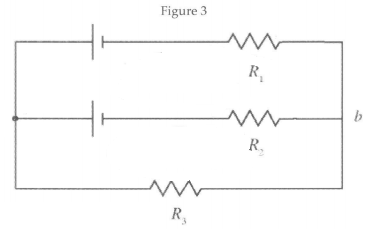# Problem: In Figure 3, consider the circuit sketched. The two batteries have negligible internal resistance and emf's  ε1 = 28.0 V and ε2 = 42.0 V. The three resistors have resistance  R1 = 2.00 Ω, R2 = 5.00 Ω, R3 = 1.00 Ω. Calculate the potential difference Va - Vb between points a and b.

🤓 Based on our data, we think this question is relevant for Professor Ford's class at TAMU.

###### Problem Details

In Figure 3, consider the circuit sketched. The two batteries have negligible internal resistance and emf's  ε1 = 28.0 V and ε2 = 42.0 V. The three resistors have resistance  R1 = 2.00 Ω, R2 = 5.00 Ω, R3 = 1.00 Ω. Calculate the potential difference Va - Vb between points a and b.